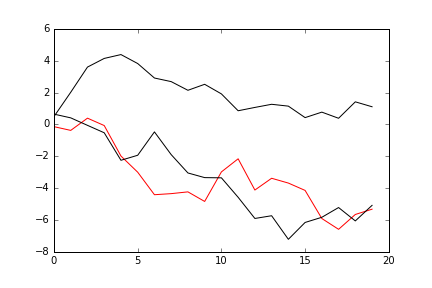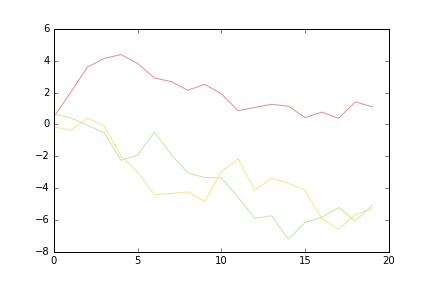### python怎么变成黑色_Matplotlib:python2的RGB颜色显示为黑色

import pandas as pd

import matplotlib.pylab as plt

import numpy as np

colors = {

‘A’: (234, 142, 142),

‘B’: (255, 224, 137),

‘C’: (189, 235, 165)}

df = pd.DataFrame(np.random.randn(20, 3), columns=list(‘ABC’)).cumsum()

fig, ax = plt.subplots()

for col in df.columns:

ax.plot(df.index.tolist(), df[col].values, color=(tuple(i/255 for i in colors[col])))

plt.show()

Python 2Python 3(确定)Python | 2.7.11 64位

IPython 4.0.3

matplotlib | 1.5.1